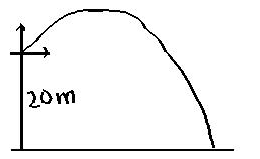Question# A 2.0 kg mass is projected from the edge of the top of a 20mtall building with a velocity of 24m/s at some unknown angle abovethe horizontal. Disregard air resistance and assume the ground islevel. What is the kinetic energy of the mass just before itstrikes the ground?

Other
ANSWEREDA 2.0 kg mass is projected from the edge of the top of a 20mtall building with a velocity of 24m/s at some unknown angle abovethe horizontal. Disregard air resistance and assume the ground islevel. What is the kinetic energy of the mass just before itstrikes the ground?2021-05-12Let the angle of projection be θ and initialvelocity be u $$\displaystyle{m}{s}^{{-{1}}}$$.
Initial vertical component of velocity $$\displaystyle={u}{\sin{{0}}}={24}{\sin{{0}}}$$
Horizontaal component of velocity $$\displaystyle={u}{\cos{{0}}}={24}{\cos{{0}}}$$
Eqn. for the vertical component: $$v_{v}^{2} = [(24\sin θ)^{2} +- (2) \times (- 9.8 m s^{-1}) \times (-20 m)]$$

$$=576 \sin^{2}\theta + 392$$

The final horizontal component: $$v_{h}^{2} = 576\cos^{2}\theta$$

K.E. when the 2 kg mass touches the ground =

$$(\frac{1}{2}) m[v_{v}^{2} +v_{h}^{2}] = 0.5 \times (2) \times (576\sin^{2}\theta + 392 + 576\cos^{2}\theta)$$

= 968 J